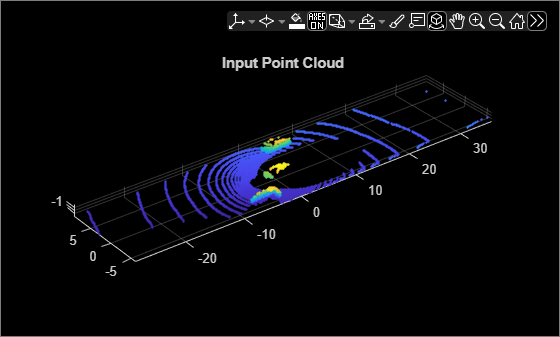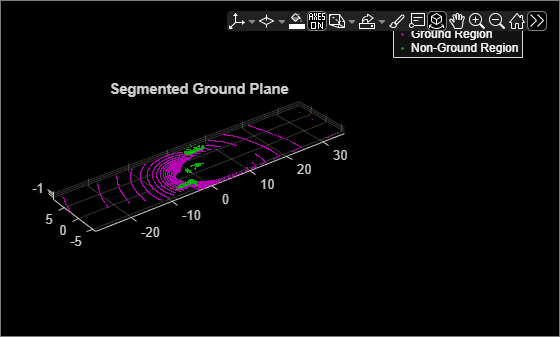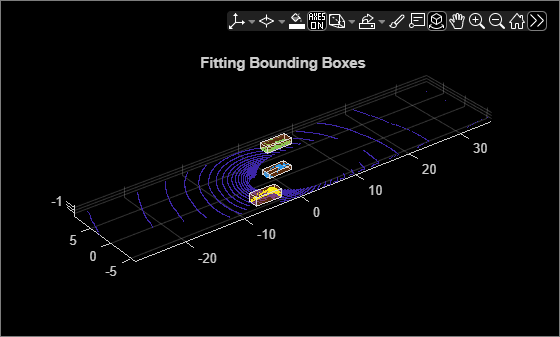# pcfitcuboid

Fit cuboid over point cloud

## Syntax

``model = pcfitcuboid(ptCloudIn)``
``model = pcfitcuboid(ptCloudIn,indices)``
``model = pcfitcuboid(___,Name,Value)``

## Description

````model = pcfitcuboid(ptCloudIn)` fits a cuboid over the input point cloud data. The function stores the properties of the cuboid in the `cuboidModel` object, `model`.```

example

````model = pcfitcuboid(ptCloudIn,indices)` fits a cuboid over a selected set of points, `indices`, in the input point cloud.```
````model = pcfitcuboid(___,Name,Value)` specifies options using one or more name-value pair arguments in addition to any of the input argument combinations in previous syntaxes. For example, ```'AzimuthRange,[-75 75]'``` sets the angular range for the azimuth angles of the function.```

## Examples

collapse all

Fit bounding boxes around clusters in a point cloud.

Load the point cloud data into the workspace.

`data = load('drivingLidarPoints.mat');`

Define and crop a region of interest (ROI) from the point cloud. Visualize the selected ROI of the point cloud.

```roi = [-40 40 -6 9 -2 1]; in = findPointsInROI(data.ptCloud,roi); ptCloudIn = select(data.ptCloud,in); hcluster = figure; panel = uipanel('Parent',hcluster,'BackgroundColor',[0 0 0]); ax = axes('Parent',panel,'Color',[0 0 0]); pcshow(ptCloudIn,'MarkerSize',30,'Parent',ax) title('Input Point Cloud')```Segment the ground plane. Visualize the segmented ground plane.

```maxDistance = 0.3; referenceVector = [0 0 1]; [~,inliers,outliers] = pcfitplane(ptCloudIn,maxDistance,referenceVector); ptCloudWithoutGround = select(ptCloudIn,outliers,'OutputSize','full'); hSegment = figure; panel = uipanel('Parent',hSegment,'BackgroundColor',[0 0 0]); ax = axes('Parent',panel,'Color',[0 0 0]); pcshowpair(ptCloudIn,ptCloudWithoutGround,'Parent',ax) legend('Ground Region','Non-Ground Region','TextColor', [1 1 1]) title('Segmented Ground Plane')```Segment the non-ground region of the point cloud into clusters. Visualize the segmented point cloud.

```distThreshold = 1; [labels,numClusters] = pcsegdist(ptCloudWithoutGround,distThreshold); labelColorIndex = labels; hCuboid = figure; panel = uipanel('Parent',hCuboid,'BackgroundColor',[0 0 0]); ax = axes('Parent',panel,'Color',[0 0 0]); pcshow(ptCloudIn.Location,labelColorIndex,'Parent',ax) title('Fitting Bounding Boxes') hold on```

Fit bounding box on each cluster, visualized as orange highlights.

```for i = 1:numClusters idx = find(labels == i); model = pcfitcuboid(ptCloudWithoutGround,idx); plot(model) end```## Input Arguments

collapse all

Point cloud, specified as a `pointCloud` object.

Indices of selected valid points, specified as a vector of positive integers.

Data Types: `single` | `double` | `int8` | `int16` | `int32` | `int64` | `uint8` | `uint16` | `uint32` | `uint64`

### Name-Value Pair Arguments

Specify optional comma-separated pairs of `Name,Value` arguments. `Name` is the argument name and `Value` is the corresponding value. `Name` must appear inside quotes. You can specify several name and value pair arguments in any order as `Name1,Value1,...,NameN,ValueN`.

Example: `'AzimuthRange',[-75 75]` sets the angular range for the azimuth angles of the function.

Range of azimuth angles over which to identify the orientation of the cuboid, specified as the comma-separated pair consisting of `'AzimuthRange'` and a two-element row vector of real values in the range [0, 90].

Data Types: `single` | `double`

Step size of search window, specified as the comma-separated pair consisting of `'Resolution'` and a positive scalar. The specified value must be less than or equal to the distance between the upper and lower bounds of the range of azimuth angles. For example, if the range of azimuth angles is [0, 90], the specified value must be less than or equal to 90.

Note

Decreasing the resolution will increase the computation time and memory footprint.

Data Types: `single` | `double`

## Output Arguments

collapse all

Cuboid model, returned as a `cuboidModel` object.

 Xiao Zhang, Wenda Xu, Chiyu Dong and John M. Dolan, "Efficient L-Shape Fitting for Vehicle Detection Using Laser Scanners", IEEE  Intelligent Vehicles Symposium, June 2018

## SupportGet trial now